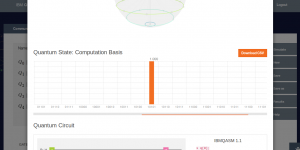# Quantum Computing – IBM Quantum CloudIn this guide, I will introduce you to the world of Quantum Computing. Let us start with the basics.

### Classical Model

Information is stored in the form of 0 and 1. All the calculations can be performed via simple logic gates.

### Quantum Model

A Quantum computer also has bits, just like any computer. But instead of 0 and 1, its quantum bits or Qubits can represent a 0 or 1 or both at once, a property known as superposition.

Now, it is not a digital computer but an analog computer.

Some of the counterintuitive ideas in Quantum Physics are :

• A physical system in a perfectly definitive state can still behave randomly.
• Two systems that are too far apart to influence each other can behave in ways that, though individually random, are somehow strongly calculated.

We’ll have a look at what the above points mean in detail.

### Quantum Entanglement

In a nutshell, an event at one point in the universe could affect another event. Something faster than light ?

All fundamental particles have a property called spin. Suppose there is an arrow door aligned with the arrow pointing upwards. If the mode of measurement is aligned with the direction of the particle spin, it is spin up or else spin down. There are 50/50 chances of spin up / down.

Now, here comes the spooky stuff : There is no well defined spin because both the particles have 50/50 chances of spin up / down and both cannot have the same spin ( Total Angular Momentum of the Universe must stay constant ). So, both of them are entangled, the spin of one is opposite to the other one. Measuring a property will tell you the other one. If an arrow door points towards left then there are chances of both of them having the same spin which is not possible. Hence, there is no predefined state.

### IBM Quantum Cloud

Have a look at : IBM Quantum Cloud

### Quantum Composer

It is a GUI for programming a Quantum Processor and allows you to make your own Quantum Circuits.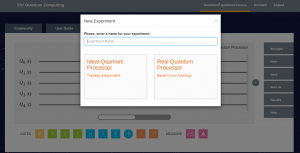You will see an option to work on either Real Processor or the Ideal Processor. According to IBM, the Ideal Processor runs your score in simulation. It permits more connections than are available with the real device which is fast and perhaps error free. Running your score in Real Processor requires units ( a pseudo currency ). You get to run your score on actual Quantum Computer. I’m going ahead with the Ideal Processor.Each line in the Composer represents a Qubit. Each Qubit has different frequency like musical notes. Quantum gates are represented by square boxes that play a frequency for different duration, amplitude and phase and are called single qubit gates.

### Measurement in The Computational Basis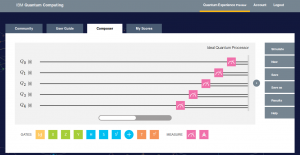Computational Basis ( Z) will measure the initial Qubit state which is 0.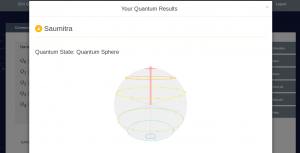As you can see, all the qubits are 0.Let us put the basis only on the first qubit and see what happens ( double click to remove gates )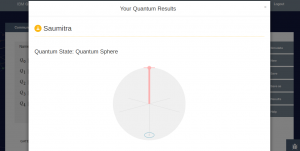Now, you see just a single qubit with a 0 because only that particular qubit is measured and not the others.I suggest you play around it for a while to get good hands on it and it goes the same for every gate.

### Pauli Gate ( X )

A bit flip which is pi rotation around the X axis. Unlike classical superposition, quantum superposition is linearly dependent on |0> and |1>.

`|0> => X|0> = |1>`

where X = [0 1; 1 0]  (Written in MATLAB format)

|0> = [1;0] and |1>= [0:1]Let us see what happens,Quantum Sphere will become clearer once we cover Bloch Measurement in the next tutorial.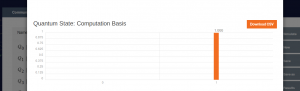Hence, we see according to the above dependencies, there is a pi rotation around x axis converting the 0 into a 1.

Let us experiment more,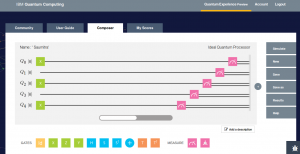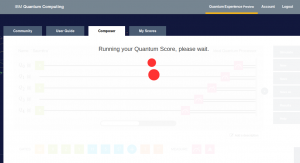The result is as expected. You can observe the pi rotation at the specified qubits. You can even download the csv file.